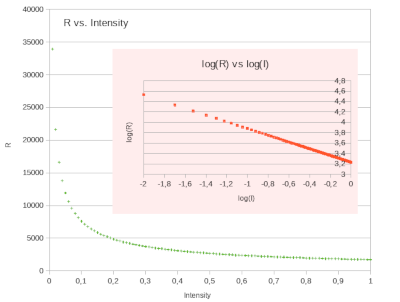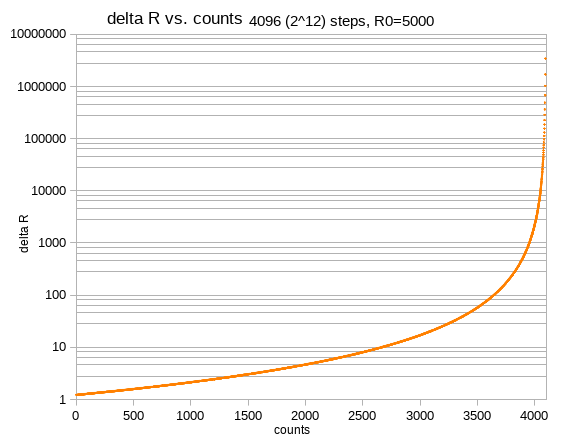# smartyPies.com

## Math: Basics

Background math for the polarimeter.

#### Ideal polarizer

The intensity of light after two polarizers with polarization planes at an angle of `phi` relative to `I_0` is described as ` I_phi = cos ^ 2 phi * I_0` Check the graph below for the dependency of intensity on the angle. You will recognize the maximums at 0°=360° and 180° and minimums at 90° and 270°. It's 180° (or `pi`)-periodic, due to the square of cosine.

#### Ideal photoresistorCdS (Cadmium Sulphide) photoresitors show an inverse dependency of resistance on incident light. The higher the luminance, the lower the resistance, ideally described as a a power function in I `R_I = c * I_phi^gamma` with `c=1700 and gamma=-0.65`. How to get the actual parameters `c` and `gamma` is hinted at in the pink graph, it will be shown with real data in the next math chapter (to come...).

#### Voltage divider

An example for a voltage divider circuit to measure the resistance of an electronic part is shown on the MCP3208 page. The fixed resistance `R_0` is connected as first in series with the variable resistance `R_I`. The formula for the resulting voltage in relation to the incoming voltage is `V_I = V_0 * (R_I /( R_0 + R_I)) = V_0 * (1 - (R_0/(R_0+R_I)))`
In case of `R_I -> 0 => V_I -> 0` and `R_I -> oo => V_I -> V_0`.

The ADC, here a MCP3208, compares an incoming voltage with a base voltage and returns the clipped ratio of these, which can also be expressed in terms of `R_0` and `R_I`, see B-3:
`counts = floor(2^p*(V_I/V_0)) = floor( 2^p * (1 -(R_0/(R_0+R_I))))`
`2^p` denotes the number of steps, with `p` being the bit depth. In case of MCP3008 `p = 10 => 1024`, for MCP3208 `p = 12 => 4096`.This graph is interesting as it shows that until ~2700 counts the change of `R_I` needed to get the next higher count value is less than 10 `Omega`, a high resolution with respect to R. After 4000 counts, the difference in R needed for the next higher counts value increases drastically. Good on the one hand, because we can determine the range of R for a certain counts value with almost no error. Bad on the other hand, because we may miss out resolution for small changes of I with low intensities.

#### Putting it together

Plugging in all formulas into one we get the dependency of counts on angle
`counts_phi= floor( 2^p*(1-(R_0/(R_0+( c*(cos^2 phi* I_0)^gamma))))`
With `I_0=1, c=1700, gamma=-0.65, p=12, R_0 in {2000, 5000, 10000}` we get following curves:#### Conclusions

• With 5kOhms resistor the counts range spreads over ~ 3000 values, while there are just 900 0.1°steps between a minimum and a maximum, e.g from 0° to 90°, suggesting a good resolution of count vs. step position.
• A higher resistance `R_0` is not recommended, as it may introduce errors due to "bleeding" currents before sampling has completed. Especially it is not advisable to put the photoresistor first in row.
• Peaks for high values (=little light) are much better defined than the valleys for low values (=much light). This is caused by big values of R for low intensities, partly compensated by the ratio in the voltage divider formula (B-3).